New update is available. Click here to update.
Last Updated: Dec 5, 2023
Easy

# Support Vector Machines

## Introduction

Classification is one of the important and very simple concepts in the field of machine learning. Whether the person is suffering or not, whether the email is spam or not, whether the credit card transaction is fraud or not, etc. These types of problems fall into the region of classification. There are many concepts and algorithms that are introduced to solve this type of problem. One of the most important and simple algorithms among them is SVM - Support Vector Machine. Similar to Logistic Regression for classification, SVM is also a classification algorithm that finds a weight vector that corresponds to a hyperplane with the largest margin that separates two classes of data. Let’s dive into the concept of SVM and learn a few important theories about it.

## SVM (Support Vector Machine)

Support Vectors are the data points that are very close to the hyperplane margin. Or we can also say that, For any hyperplane, support vectors are the data points that define the hyperplane margin. For Example: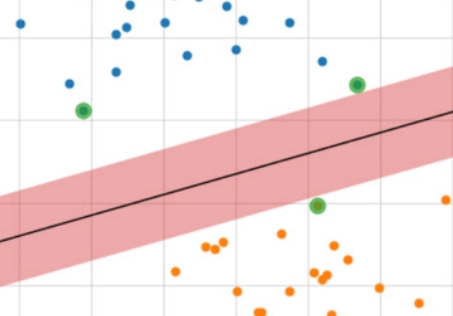In the above example, we can say that the green-colored highlighted points are considered to support vectors.
The objective of SVM is to perform a classification task using a liner indicator function i.e., a hyperplane whose margin is as large as possible. Example: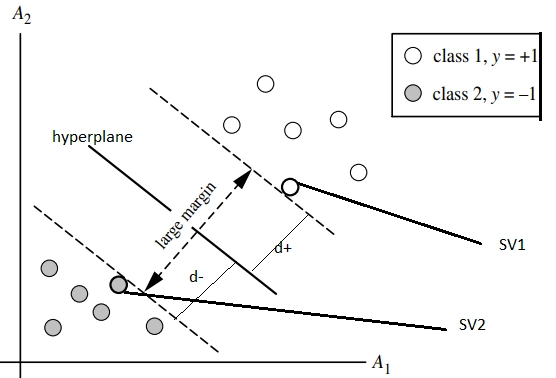SVMs can be used for both classification tasks and regression tasks. Let’s solely focus on classification tasks and understand how they work.
In the case of classification tasks, two types of datasets will be present. They are 1. Linearly separable dataset. 2. Linearly Inseparable dataset.

## SVMs for Linearly Separable Classes

In the two-class classification problem, we are given an input dataset containing two classes of data and an indicator function to map the data into classes. Say from the above figure, class 1 as C1 contains a set of positive samples whose indicator function value is +1, and class 2 as C2 contains a set of negative samples whose indicator function value is -1.
Then we try to find a linearly separable function, a hyperplane which is defined as
f(W, X, wo) = W.X+wo  ,
where W = set of weights defined on the input

X = input values

w= bias or a constant.

Let d+ and d- are the perpendicular distances from separating hyperplane (margin lines) which are closed to data points. The distance of margin is defined as
d+ = W.Xi + w >= 1, for di = +1 for C1
d-  = W.Xi + w <= -1, for di = -1 for C2

Let’s derive the margin:
We have SV1 (say X+) and SV2 (say Xπ) as support vectors. Then, we have
W.X+ + w = 1 for C1 and similarly
W.Xπ + w = 0 for C2.
Then from the above equations, we have W(X+ - Xπ) = 1
This above equation can be written as W( ||X+ - Xπ||. W / ||W||) = 1 => ||X+ - Xπ||. ||W||2 / ||W|| = 1
At last, we get ||X+ - Xπ|| . ||W|| = 1  => ||X+ - Xπ|| = 1 / ||W||
Therefore, we can tell that the perpendicular distance will be concluded as d+ = d- = 1 / ||W||
Therefore total margin can be calculated as M = 2 / ||W||.
This can be diagrammatically shown below.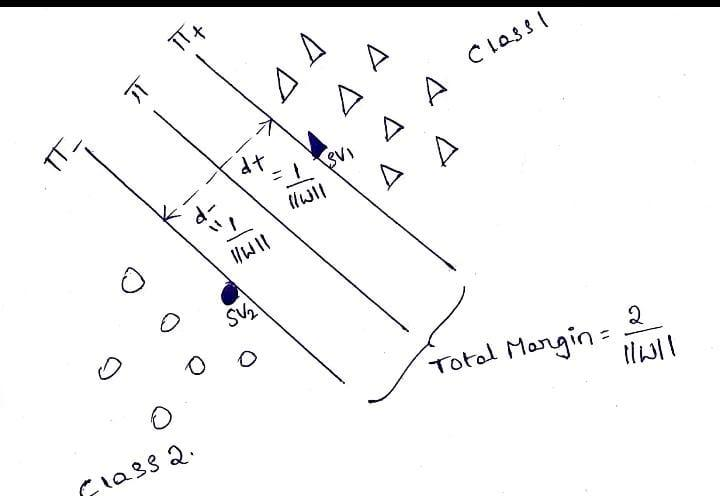That's it. We find a hyperplane that separates two-class data points with a large margin.
Let’s have a look at the linearly inseparable case.

## SVMs for Linearly Inseparable Classes

In the case of the non-separable dataset, the points of opposite classes may overlap. In this case, the constraints di(W.X + wo) >= 1, for i = 1,2,.... cannot be satisfied for all data points.
Or we can also say that when the data points are scattered across the input space, identifying the margin line takes more time and more repetitions. Then, those data are called linearly non-separable data. To separate these data, we need to identify a soft margin that separates data in its most accurate way.
Here we actually introduce a new slack variable to the class equations. Then we have
W.Xi + w >= 1 - 𝛏, di = +1
W.Xi + w <= -1 + 𝛏, di = -1 here the additional 𝛏 variable is called a slack variable, a constant. Here we can say the introduction of the slack variable makes the soft margin classifier loss as a hinge loss.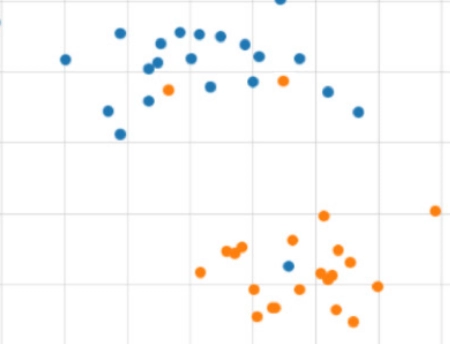Linearly Inseparable Data

Then by doing the same process, we did for Linearly separable data to find the total margin, we can get a value of

M = 2( 1 - 2𝛏 ) / ||W||

There are more interesting features about SVMs, like using kernels in SVM. This will be a really a broad topic to understand. Basically, these kernel functions are introduced so as to replace the dot function scenarios. Three admissible functions are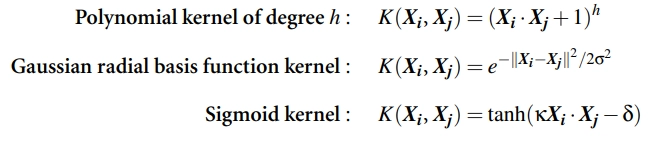We will cover this concept later in our articles.

## SVMs using scikit learn Python

Python’s scikit-learn library provides three ways of implementing the SVM classifier.
They are

1. svm.LinearSVC
2. svm.SVC ->gives an advantage of using kernels.
3. linear_model.SGDClassifier

You can go through these concepts in more detail through their official documentation linked above.

• SVMs can also be used for linearly Inseparable data using the kernel support.
• It can also be used in the case of high dimensionality and even when the number of data points is smaller than its dimensionality.
• It can also be used for Clustering(linearly Inseparable), Classification, and Regression problems.
• Works well with even structured and unstructured data like text, images, etc.
• The only disadvantage is it won't work well on large datasets when the dataset has more noise.

## Implement SVM in Python

Code: Here, I will use a small example to demonstrate how SVMs are used to classification problems. We have used sci-kit and few other libraries for this. Let’s get to code:

``````import numpy as np
X = np.array([[-1, -1], [-2, -1], [1, 1], [2, 1]])
y = np.array([1, 1, 2, 2])``````

In the above three lines of code, we have defined two NumPy arrays. X has the points and Y has the classes to which these points belong to. Now, let us create our SVM model using sklearn.svm. Here, I choose the linear kernel.

``````from sklearn.svm import SVC
clf = SVC(kernel='linear')``````

We now fit out classifier(clf) to the data points we defined.
clf.fit(X, y)

To predict the class of a new dataset

prediction = clf.predict([[0,6]])

This would return us the prediction(a class to which the data belongs). Voila! It is simple to use an SVM for simple classification problems.

## Here are some real-world applications of support vector machines listed

1. Face Detection: SVM separates parts of the image as facial and non-facial and forms a square border around the face.

2. Text and Hypertext Categorisation: SVMs allow text and hypertext categorisation for both inductive and transductive models. They use training data to classify documents into different categories. It categorises on the basis of the score generated and then compares with the threshold value.

3. Classification of images: We have already discussed that SVMs are widely used in image classification problems. It provides better accuracy for image classification and image search in comparison to the formerly used query-based searching approaches.

4. Bioinformatics: SVMs are really popular in medical and bioinformatics and are used in protein classification and cancer classification problems. It is used for identifying the classification of genes, patients on the basis of genes, and other biological problems like skin cancer.

5. Protein fold and remote homology detection: SVM algorithms are also widely used in protein remote homology detection.

6. Handwriting recognition: SVMs are used to recognise handwritten characters and work with a wide variety of languages.

7. Generalised Predictive Control (GPC): Use SVM based GPC to control chaotic dynamics with useful parameters and hyperparameters.

### What does SVM stand for?

SVM stands for support vector machine. Basically, SVMs or support vector machines are used for both classification and regression tasks. It mainly saves the complexity. It improves the performance of tasks by implementing its kernel trick.

### What is an SVM kernel?

An SVM kernel is a trick that is used by the support vector machine algorithm to improve its performance. These kernels are used to transform the input data space into the required form. For example, a kernel may take a low dimensional input space and transform it into a higher dimensional data space.

### What is the agenda in the SVM concept?

The objective of the SVM is to perform classification and regression tasks by finding a linear indicator function, a hyperplane that separates two classes.

### What are the advantages of using SVM?

SVMs are mainly used to reduce complexity. It can be used for both linearly separable and non-separable, for both classification and regression, and for structured and unstructured datasets.

## Conclusion

So far, we have discussed the concept of SVM and how it works. The math behind this concept. We will learn the concept of kernels in SVM in the upcoming articles. Until then,  keep exploring.
Hey Ninjas! You can check and explore more unique courses on machine learning concepts through our official website, Coding Ninjas, and checkout Coding Ninjas Studio to learn through articles and other important stuff to your growth.
Happy Learning!